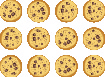Dividing Whole Numbers: Notation and Modeling

Learning Outcomes

• Use words and symbols to represent division
• Model division of whole numbers using base-10 blocks

Use Division Notation

So far we have explored addition, subtraction, and multiplication. Now let’s consider division. Suppose you have the $12$ cookies and want to package them in bags with $4$ cookies in each bag. How many bags would we need?You might put $4$ cookies in first bag, $4$ in the second bag, and so on until you run out of cookies. Doing it this way, you would fill $3$ bags.In other words, starting with the $12$ cookies, you would take away, or subtract, $4$ cookies at a time. Division is a way to represent repeated subtraction just as multiplication represents repeated addition.

Instead of subtracting $4$ repeatedly, we can write

$12\div 4$

We read this as twelve divided by four and the result is the quotient of $12$ and $4$. The quotient is $3$ because we can subtract $4$ from $12$ exactly $3$ times. We call the number being divided the dividend and the number dividing it the divisor. In this case, the dividend is $12$ and the divisor is $4$.

In the past you may have used the notation $4\overline{)12}$ , but this division also can be written as $12\div 4, 12\text{/}4, \frac{12}{4}$. In each case the $12$ is the dividend and the $4$ is the divisor.

Operation Symbols for Division

To represent and describe division, we can use symbols and words.

Operation Notation Expression Read as Result
$\text{Division}$ $a \div b$

$\frac{a}{b}$

$b\overline{)a}$

$a/b$

$12\div 4$

$\frac{12}{4}$

$4\overline{)12}$

$12/4$

$\text{Twelve divided by four}$ $\text{the quotient of 12 and 4}$

Division is performed on two numbers at a time. When translating from math notation to English words, or English words to math notation, look for the words of and and to identify the numbers.

example

Translate from math notation to words.
1. $64\div 8$

2. $\frac{42}{7}$

3. $4\overline{)28}$

Solution

1. We read this as sixty-four divided by eight and the result is the quotient of sixty-four and eight.
2. We read this as forty-two divided by seven and the result is the quotient of forty-two and seven.
3. We read this as twenty-eight divided by four and the result is the quotient of twenty-eight and four.

try it

In the video below we present more examples of how to express division with words, and how to translate those words into math notation.

Model Division of Whole Numbers

As we did with multiplication, we will model division using counters. The operation of division helps us organize items into equal groups as we start with the number of items in the dividend and subtract the number in the divisor repeatedly.

Doing the Manipulative Mathematics activity Model Division of Whole Numbers will help you develop a better understanding of dividing whole numbers.

example

Model the division: $24\div 8$

try it

Model: $24\div 6$

Model: $42\div 7$

In the video below we show another way to model division using area.